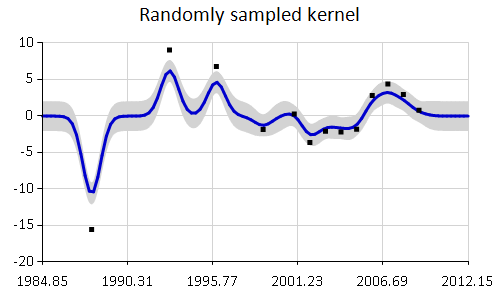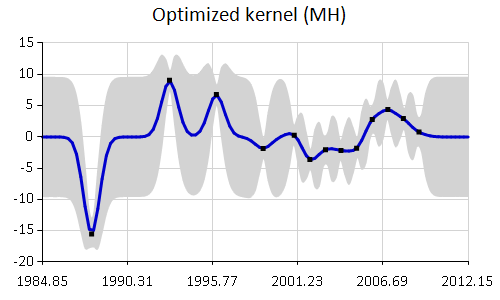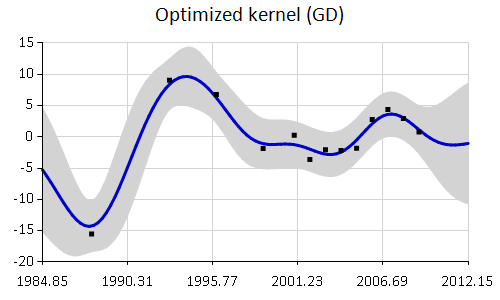# Optimization

This section shows how to optimize hyperparameters of covariance functions in Ariadne. The package currently implements two methods:

• Metropolis-Hastings algorithm for sampling from the posterior. This method requires specifying a prior distribution for each hyperparameter.

• Simple gradient descent algorithm. The implementation includes only a very basic form of gradient descent.

## Metropolis-Hastings algorithm

The Metropolis-Hastings algorithm is a simple Monte Carlo method for sampling from the posterior distribution. Let $$\theta$$ represent hyperparameters of a covariance function. Then the posterior distribution is defined as

$p(\theta \mid X, Y) \propto p(Y \mid X, \theta) p(\theta)$

where $$X$$ are locations of observations and $$Y$$ are the observed function values. Then $$p(Y \mid X, \theta)$$ is the Gaussian process likelihood of observed data.

To be able to sample from the posterior distribution, first we need to specify a prior distribution over hyperparameters $$p(\theta)$$. For the squared covariance function, hyperparameters are the lengthscale $$l$$, signal variance $$\sigma^2$$ and noise variance $$\sigma^2_{\text{noise}}$$.

$\theta = \left( l, \sigma^2, \sigma^2_{\text{noise}} \right)$

### Specifying prior distribution

Because the hyperparameters must be positive, we will use a simple log-normal prior distribution for each hyperparameter.

  1: 2: 3: 4: 5: 6: 7: 8: 9: 10: 11:  #r "Ariadne.dll" open Ariadne.GaussianProcess open Ariadne.Kernels #r "MathNet.Numerics.dll" open MathNet.Numerics let rnd = System.Random(3) let lengthscalePrior = Distributions.LogNormal.WithMeanVariance(2.0, 4.0, rnd) let variancePrior = Distributions.LogNormal.WithMeanVariance(1.0, 5.0, rnd) let noisePrior = Distributions.LogNormal.WithMeanVariance(0.5, 1.0, rnd)

Ariadne includes a simplified method to work with squared exponential kernels. We can define a prior distribution over squared covariance hyperparameters and directly sample squared covariance kernels from it.

 1: 2: 3:  let prior = SquaredExp.Prior(lengthscalePrior, variancePrior, noisePrior) let kernel = prior.Sample() let gp = kernel.GaussianProcess()

Now that we specified a prior distribution, we can use Metropolis-Hastings algorithm to get samples from the posterior distribution of hyperparameters given training data.

 1: 2: 3: 4: 5: 6:  // Optimization open Ariadne.Optimization let data = [{Locations = (...) Observations = (...) }]

To sample from the posterior distribution with squared exponential kernel, we can use a built-in function optimizeMetropolis. This function runs Metropolis-Hastings algorithm with symmetric proposal distribution, i.e. basic Metropolis algorithm.

  1: 2: 3: 4: 5: 6: 7: 8: 9: 10: 11: 12: 13: 14: 15: 16:  // Metropolis sampling for squared exponential with default settings let newKernel1 = kernel |> SquaredExp.optimizeMetropolis data MetropolisHastings.defaultSettings prior // Specify custom parameters open Ariadne.Optimization.MetropolisHastings let settings = { Burnin = 500 // Number of burn-in iterations Lag = 5 // Thinning of posterior samples SampleSize = 100 } // Number of thinned posterior samples let newKernel2 = kernel |> SquaredExp.optimizeMetropolis data settings prior

The Metropolis sampler takes a specified total number of samples, 50 by default, and returns the posterior mean estimate for each hyperparameter.

Squared exponential, l = 0.52, σ² = 22.41, σ²_{noise} = 0.26


We can compare difference in fit of the randomly sampled covariance parameters kernel and sampled values in newKernel2. You might need to re-run the sampler for more iterations or with a different starting location to arrive to a good posterior estimate of hyperparameter values. Note that each iteration of the Metropolis-Hastings sampler has $$\mathcal{O}(N^3)$$ time complexity.

 1: 2: 3: 4: 5: 6:  let gpInit = kernel.GaussianProcess() let gpOptim = newKernel2.GaussianProcess() let loglikInit = gpInit.LogLikelihood data let loglikOptim = gpOptim.LogLikelihood data printfn "Initial log likelihood: %f \nFinal log likelihood: %f" loglikInit loglikOptim
Initial log likelihood: -231.973587
Final log likelihood: -41.512115


 1: 2: 3: 4: 5:  open FSharp.Charting kernel.GaussianProcess() |> plot data |> Chart.WithTitle("Randomly sampled kernel", InsideArea=false) |> Chart.WithMargin(0.0,10.0,0.0,0.0)1: 2: 3:  newKernel2.GaussianProcess() |> plot data |> Chart.WithTitle("Optimized kernel (MH)", InsideArea=false) |> Chart.WithMargin(0.0,10.0,0.0,0.0)### Running general Metropolis-Hastings

It is also possible to run general Metropolis-Hastings algorithm to obtain samples from the posterior distribution by calling sampleMetropolisHastings function. The function operates over an array of parameter values. As parameters, it takes functions that compute log likelihood, transition probability, Markov Chain settings and a proposal sampler. See the source code for details.

It is also possible to fit hyperparameters of covariance functions using any gradient-based optimization algorithm. Ariadne currently implements only basic gradient descent.

The gradientDescent function operates over an array of parameter values. It requires a function that computes gradient of log likelihood with respect to all hyperparameters. There is an implementation of gradient for squared exponential covariance kernel. Because there are local maxima in the likelihood function, gradient descent might require several restarts with different initial locations and step sizes.

  1: 2: 3: 4: 5: 6: 7: 8: 9: 10: 11: 12: 13: 14:  // Gradient descent settings let gdSettings = { GradientDescent.Iterations = 10000; GradientDescent.StepSize = 0.01} let gradientFunction parameters = SquaredExp.fullGradient data parameters // Run gradient descent let kernelGD = gradientDescent gradientFunction gdSettings (kernel.Parameters) |> SquaredExp.ofParameters let gpGD = kernelGD.GaussianProcess() printfn "Original Gaussian process likelihood: %f" (gpInit.LogLikelihood data) printfn "Optimized Gaussian process likelihood: %f" (gpGD.LogLikelihood data)
Original Gaussian process likelihood: -231.973587
Optimized Gaussian process likelihood: -37.614942


 1: 2: 3:  gpGD |> plot data |> Chart.WithTitle("Optimized kernel (GD)", InsideArea=false) |> Chart.WithMargin(0.0,10.0,0.0,0.0)module GaussianProcess

module Kernels

namespace MathNet
namespace MathNet.Numerics
val rnd : System.Random

Full name: Optimization.rnd
namespace System
Multiple items
type Random =
new : unit -> Random + 1 overload
member Next : unit -> int + 2 overloads
member NextBytes : buffer:byte[] -> unit
member NextDouble : unit -> float

Full name: System.Random

--------------------
System.Random() : unit
System.Random(Seed: int) : unit
val lengthscalePrior : Distributions.LogNormal

Full name: Optimization.lengthscalePrior
namespace MathNet.Numerics.Distributions
Multiple items
type LogNormal =
new : mu:float * sigma:float -> LogNormal + 1 overload
member CumulativeDistribution : x:float -> float
member Density : x:float -> float
member DensityLn : x:float -> float
member Entropy : float
member InverseCumulativeDistribution : p:float -> float
member Maximum : float
member Mean : float
member Median : float
member Minimum : float
...

Full name: MathNet.Numerics.Distributions.LogNormal

--------------------
Distributions.LogNormal(mu: float, sigma: float) : unit
Distributions.LogNormal(mu: float, sigma: float, randomSource: System.Random) : unit
Distributions.LogNormal.WithMeanVariance(mean: float, var: float, ?randomSource: System.Random) : Distributions.LogNormal
val variancePrior : Distributions.LogNormal

Full name: Optimization.variancePrior
val noisePrior : Distributions.LogNormal

Full name: Optimization.noisePrior
val prior : SquaredExp.Prior

Full name: Optimization.prior
module SquaredExp

Multiple items
type Prior =
new : lengthscalePrior:LogNormal * signalVariancePrior:LogNormal * noiseVariancePrior:LogNormal -> Prior
member DensityLn : se:SquaredExp -> float
member ParamsDensityLn : parameters:float [] -> float
member Sample : unit -> SquaredExp
member LengthscalePrior : LogNormal
member NoiseVariancePrior : LogNormal
member SignalVariancePrior : LogNormal

--------------------
new : lengthscalePrior:Distributions.LogNormal * signalVariancePrior:Distributions.LogNormal * noiseVariancePrior:Distributions.LogNormal -> SquaredExp.Prior
val kernel : SquaredExp.SquaredExp

Full name: Optimization.kernel
member SquaredExp.Prior.Sample : unit -> SquaredExp.SquaredExp
val gp : GaussianProcess<float>

Full name: Optimization.gp
member SquaredExp.SquaredExp.GaussianProcess : unit -> GaussianProcess<float>
module Optimization

val data : Observation<float> list

Full name: Optimization.data
[|1988.0; 1993.0; 1996.0; 1999.0; 2001.0; 2002.0; 2003.0; 2004.0; 2005.0;
2006.0; 2007.0; 2008.0; 2009.0|];
[|-15.52307692; 9.056923077; 6.786923077; -1.843076923; 0.2769230769;
-3.623076923; -2.063076923; -2.183076923; -1.813076923; 2.806923077;
4.386923077; 2.946923077; 0.7869230769|];
val newKernel1 : SquaredExp.SquaredExp

Full name: Optimization.newKernel1
Multiple items
module SquaredExp

--------------------
module SquaredExp

val optimizeMetropolis : data:seq<Observation<float>> -> settings:MetropolisHastings.Settings -> prior:SquaredExp.Prior -> initialKernel:SquaredExp.SquaredExp -> SquaredExp.SquaredExp

module MetropolisHastings

val defaultSettings : MetropolisHastings.Settings

val settings : Settings

Full name: Optimization.settings
val newKernel2 : SquaredExp.SquaredExp

Full name: Optimization.newKernel2
val optimizeMetropolis : data:seq<Observation<float>> -> settings:Settings -> prior:SquaredExp.Prior -> initialKernel:SquaredExp.SquaredExp -> SquaredExp.SquaredExp

val gpInit : GaussianProcess<float>

Full name: Optimization.gpInit
val gpOptim : GaussianProcess<float>

Full name: Optimization.gpOptim
val loglikInit : float

Full name: Optimization.loglikInit
member GaussianProcess.LogLikelihood : data:seq<Observation<'T>> -> float
val loglikOptim : float

Full name: Optimization.loglikOptim
val printfn : format:Printf.TextWriterFormat<'T> -> 'T

Full name: Microsoft.FSharp.Core.ExtraTopLevelOperators.printfn
namespace FSharp
namespace FSharp.Charting
val plot : data:seq<Observation<float>> -> gp:GaussianProcess<float> -> ChartTypes.GenericChart

type Chart =
static member Area : data:seq<#value> * ?Name:string * ?Title:string * ?Labels:#seq<string> * ?Color:Color * ?XTitle:string * ?YTitle:string -> GenericChart
static member Area : data:seq<#key * #value> * ?Name:string * ?Title:string * ?Labels:#seq<string> * ?Color:Color * ?XTitle:string * ?YTitle:string -> GenericChart
static member Bar : data:seq<#value> * ?Name:string * ?Title:string * ?Labels:#seq<string> * ?Color:Color * ?XTitle:string * ?YTitle:string -> GenericChart
static member Bar : data:seq<#key * #value> * ?Name:string * ?Title:string * ?Labels:#seq<string> * ?Color:Color * ?XTitle:string * ?YTitle:string -> GenericChart
static member BoxPlotFromData : data:seq<#key * #seq<'a2>> * ?Name:string * ?Title:string * ?Color:Color * ?XTitle:string * ?YTitle:string * ?Percentile:int * ?ShowAverage:bool * ?ShowMedian:bool * ?ShowUnusualValues:bool * ?WhiskerPercentile:int -> GenericChart (requires 'a2 :> value)
static member BoxPlotFromStatistics : data:seq<#key * #value * #value * #value * #value * #value * #value> * ?Name:string * ?Title:string * ?Labels:#seq<string> * ?Color:Color * ?XTitle:string * ?YTitle:string * ?Percentile:int * ?ShowAverage:bool * ?ShowMedian:bool * ?ShowUnusualValues:bool * ?WhiskerPercentile:int -> GenericChart
static member Bubble : data:seq<#value * #value> * ?Name:string * ?Title:string * ?Labels:#seq<string> * ?Color:Color * ?XTitle:string * ?YTitle:string * ?BubbleMaxSize:int * ?BubbleMinSize:int * ?BubbleScaleMax:float * ?BubbleScaleMin:float * ?UseSizeForLabel:bool -> GenericChart
static member Bubble : data:seq<#key * #value * #value> * ?Name:string * ?Title:string * ?Labels:#seq<string> * ?Color:Color * ?XTitle:string * ?YTitle:string * ?BubbleMaxSize:int * ?BubbleMinSize:int * ?BubbleScaleMax:float * ?BubbleScaleMin:float * ?UseSizeForLabel:bool -> GenericChart
static member Candlestick : data:seq<#value * #value * #value * #value> * ?Name:string * ?Title:string * ?Labels:#seq<string> * ?Color:Color * ?XTitle:string * ?YTitle:string -> CandlestickChart
static member Candlestick : data:seq<#key * #value * #value * #value * #value> * ?Name:string * ?Title:string * ?Labels:#seq<string> * ?Color:Color * ?XTitle:string * ?YTitle:string -> CandlestickChart
...

Full name: FSharp.Charting.Chart
static member Chart.WithTitle : ?Text:string * ?InsideArea:bool * ?Style:ChartTypes.TextStyle * ?FontName:string * ?FontSize:float * ?FontStyle:System.Drawing.FontStyle * ?Background:ChartTypes.Background * ?Color:System.Drawing.Color * ?BorderColor:System.Drawing.Color * ?BorderWidth:int * ?BorderDashStyle:ChartTypes.DashStyle * ?Orientation:ChartTypes.TextOrientation * ?Alignment:System.Drawing.ContentAlignment * ?Docking:ChartTypes.Docking -> ('a0 -> 'a0) (requires 'a0 :> ChartTypes.GenericChart)
static member Chart.WithMargin : left:float * top:float * right:float * bottom:float -> ('T -> 'T) (requires 'T :> ChartTypes.GenericChart)

Full name: Optimization.gdSettings

val gradientFunction : parameters:float [] -> float []

val parameters : float []
val fullGradient : data:seq<Observation<float>> -> parameters:float [] -> float []

val kernelGD : SquaredExp.SquaredExp

Full name: Optimization.kernelGD